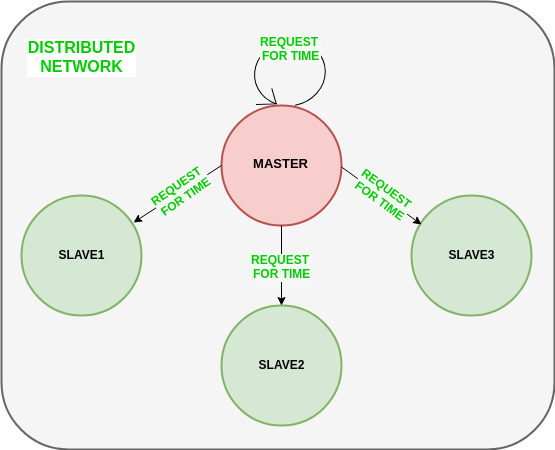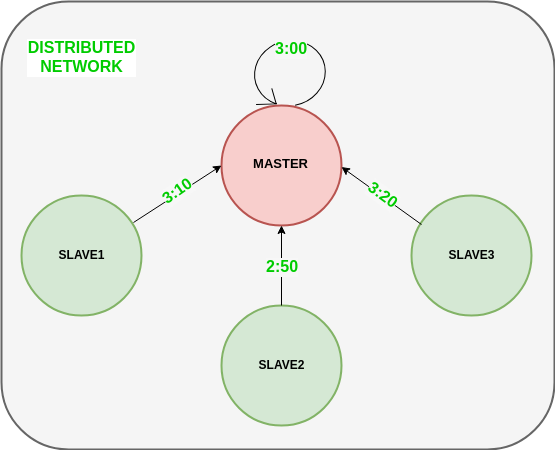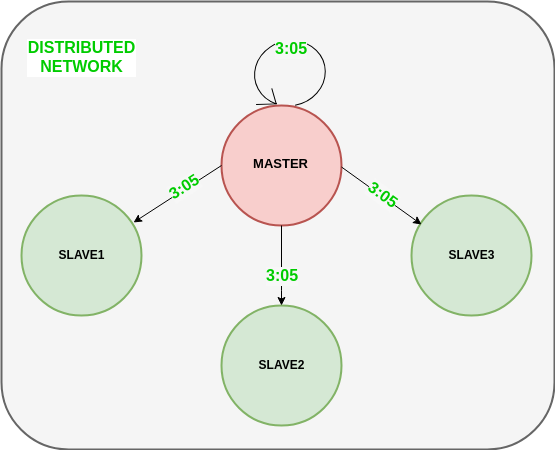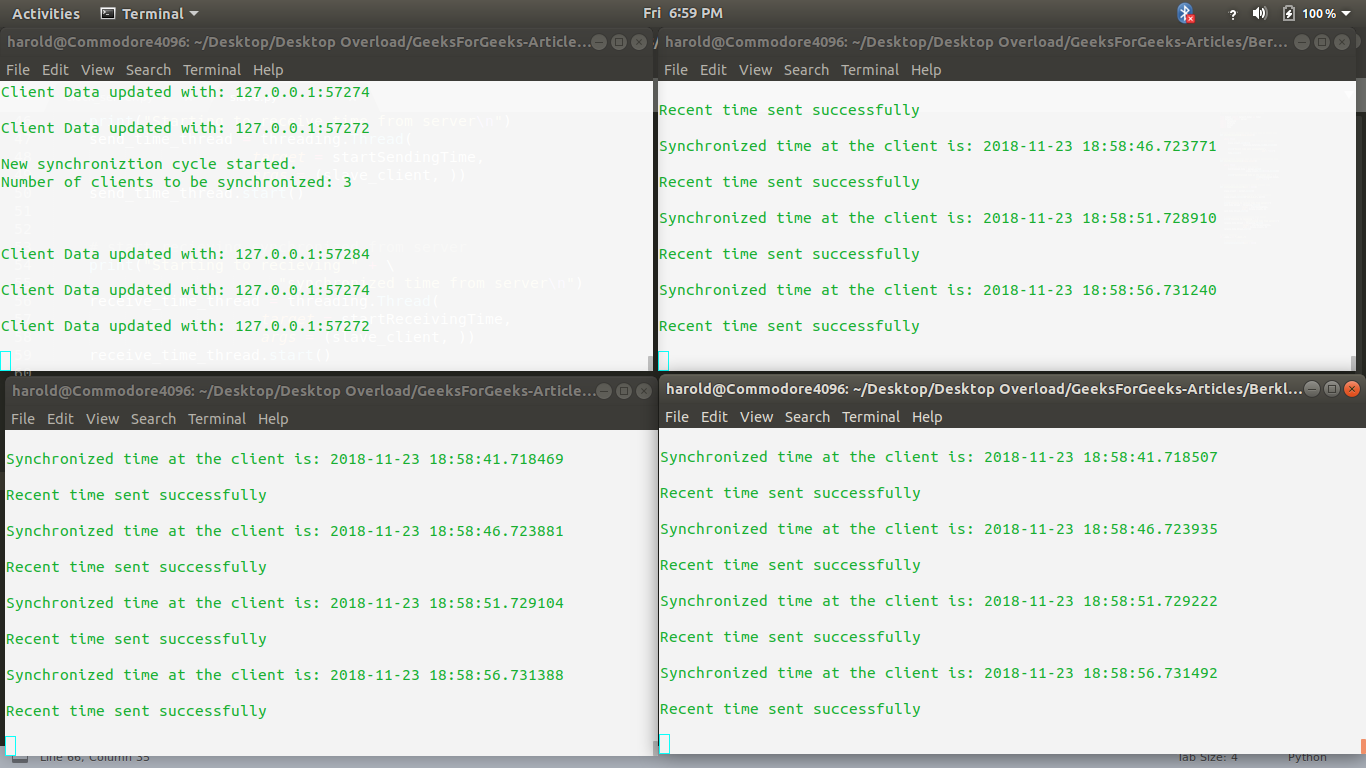# Berkeley’s Algorithm

Berkeley’s Algorithm is a clock synchronization technique used in distributed systems. The algorithm assumes that each machine node in the network either doesn’t have an accurate time source or doesn’t possess an UTC server.

Algorithm
1) An individual node is chosen as the master node from a pool nodes in the network. This node is the main node in the network which acts as a master and rest of the nodes act as slaves. Master node is chosen using a election process/leader election algorithm.

2) Master node periodically pings slaves nodes and fetches clock time at them using Cristian’s algorithm.

Diagram below illustrates how the master sends request to slave nodes.Diagram below illustrates how slave nodes send back time given by their system clock.3) Master node calculates average time difference between all the clock times received and the clock time given by master’s system clock itself. This average time difference is added to the current time at master’s system clock and broadcasted over the network.

Psuedocode for above step:

``````# receiving time from all slave nodes
repeat_for_all_slaves:
# calculating time difference
time_difference = time_at_master_node - time_at_slave_node

# average time difference calculation
average_time_difference = sum(all_time_differences) / number_of_slaves

synchronized_time = current_master_time + average_time_difference

# broadcasting synchronized to whole network
``````

Diagram below illustrates the last step of Berkeley’s algorithm.Scope of Improvement

• Improvision in accuracy of cristian’s algorithm.
• Ignoring significant outliers in calculation of average time difference
• In case master node fails/corrupts, a secondary leader must be ready/pre-chosen to take the place of the master node to reduce downtime caused due to master’s unavailability.
• Instead of sending the synchronized time, master broadcasts relative inverse time difference, which leads to decrease in latency induced by traversal time in the network while time of calculation at slave node.

The code below is a python script which can be used to trigger a master clock server.

 `# Python3 program imitating a clock server ` ` `  `from` `functools ``import` `reduce` `from` `dateutil ``import` `parser ` `import` `threading ` `import` `datetime ` `import` `socket ` `import` `time ` ` `  ` `  `# datastructure used to store client address and clock data ` `client_data ``=` `{} ` ` `  ` `  `''' nested thread function used to receive  ` `    ``clock time from a connected client '''` `def` `startRecieveingClockTime(connector, address): ` ` `  `    ``while` `True``: ` `        ``# recieve clock time ` `        ``clock_time_string ``=` `connector.recv(``1024``).decode() ` `        ``clock_time ``=` `parser.parse(clock_time_string) ` `        ``clock_time_diff ``=` `datetime.datetime.now() ``-` `\ ` `                                                 ``clock_time ` ` `  `        ``client_data[address] ``=` `{ ` `                       ``"clock_time"`      `: clock_time, ` `                       ``"time_difference"` `: clock_time_diff, ` `                       ``"connector"`       `: connector ` `                       ``} ` ` `  `        ``print``(``"Client Data updated with: "``+` `str``(address), ` `                                              ``end ``=` `"\n\n"``) ` `        ``time.sleep(``5``) ` ` `  ` `  `''' master thread function used to open portal for  ` `    ``accepting clients over given port '''` `def` `startConnecting(master_server): ` `     `  `    ``# fetch clock time at slaves / clients ` `    ``while` `True``: ` `        ``# accepting a client / slave clock client ` `        ``master_slave_connector, addr ``=` `master_server.accept() ` `        ``slave_address ``=` `str``(addr[``0``]) ``+` `":"` `+` `str``(addr[``1``]) ` ` `  `        ``print``(slave_address ``+` `" got connected successfully"``) ` ` `  `        ``current_thread ``=` `threading.Thread( ` `                         ``target ``=` `startRecieveingClockTime, ` `                         ``args ``=` `(master_slave_connector, ` `                                           ``slave_address, )) ` `        ``current_thread.start() ` ` `  ` `  `# subroutine function used to fetch average clock difference ` `def` `getAverageClockDiff(): ` ` `  `    ``current_client_data ``=` `client_data.copy() ` ` `  `    ``time_difference_list ``=` `list``(client[``'time_difference'``]  ` `                                ``for` `client_addr, client  ` `                                    ``in` `client_data.items()) ` `                                    `  ` `  `    ``sum_of_clock_difference ``=` `sum``(time_difference_list, \ ` `                                   ``datetime.timedelta(``0``, ``0``)) ` ` `  `    ``average_clock_difference ``=` `sum_of_clock_difference \ ` `                                         ``/` `len``(client_data) ` ` `  `    ``return`  `average_clock_difference ` ` `  ` `  `''' master sync thread function used to generate  ` `    ``cycles of clock synchronization in the network '''` `def` `synchronizeAllClocks(): ` ` `  `    ``while` `True``: ` ` `  `        ``print``(``"New synchroniztion cycle started."``) ` `        ``print``(``"Number of clients to be synchronized: "` `+` `\ ` `                                     ``str``(``len``(client_data))) ` ` `  `        ``if` `len``(client_data) > ``0``: ` ` `  `            ``average_clock_difference ``=` `getAverageClockDiff() ` ` `  `            ``for` `client_addr, client ``in` `client_data.items(): ` `                ``try``: ` `                    ``synchronized_time ``=` `\ ` `                         ``datetime.datetime.now() ``+` `\ ` `                                    ``average_clock_difference ` ` `  `                    ``client[``'connector'``].send(``str``( ` `                               ``synchronized_time).encode()) ` ` `  `                ``except` `Exception as e: ` `                    ``print``(``"Something went wrong while "` `+` `\ ` `                          ``"sending synchronized time "` `+` `\ ` `                          ``"through "` `+` `str``(client_addr)) ` ` `  `        ``else` `: ` `            ``print``(``"No client data."` `+` `\ ` `                        ``" Synchronization not applicable."``) ` ` `  `        ``print``(``"\n\n"``) ` ` `  `        ``time.sleep(``5``) ` ` `  ` `  `# function used to initiate the Clock Server / Master Node ` `def` `initiateClockServer(port ``=` `8080``): ` ` `  `    ``master_server ``=` `socket.socket() ` `    ``master_server.setsockopt(socket.SOL_SOCKET, ` `                                   ``socket.SO_REUSEADDR, ``1``) ` ` `  `    ``print``(``"Socket at master node created successfully\n"``) ` `       `  `    ``master_server.bind(('', port)) ` ` `  `    ``# Start listening to requests ` `    ``master_server.listen(``10``) ` `    ``print``(``"Clock server started...\n"``) ` ` `  `    ``# start making connections ` `    ``print``(``"Starting to make connections...\n"``) ` `    ``master_thread ``=` `threading.Thread( ` `                        ``target ``=` `startConnecting, ` `                        ``args ``=` `(master_server, )) ` `    ``master_thread.start() ` ` `  `    ``# start synchroniztion ` `    ``print``(``"Starting synchronization parallely...\n"``) ` `    ``sync_thread ``=` `threading.Thread( ` `                          ``target ``=` `synchronizeAllClocks, ` `                          ``args ``=` `()) ` `    ``sync_thread.start() ` ` `  ` `  ` `  `# Driver function ` `if` `__name__ ``=``=` `'__main__'``: ` ` `  `    ``# Trigger the Clock Server ` `    ``initiateClockServer(port ``=` `8080``) `

Output:

```New synchroniztion cycle started.
Number of clients to be synchronized: 3

Client Data updated with: 127.0.0.1:57284

Client Data updated with: 127.0.0.1:57274

Client Data updated with: 127.0.0.1:57272
```

The code below is a python script which can be used to trigger a slave/client.

 `# Python3 program imitating a client process ` ` `  `from` `timeit ``import` `default_timer as timer ` `from` `dateutil ``import` `parser ` `import` `threading ` `import` `datetime ` `import` `socket  ` `import` `time ` ` `  ` `  `# client thread function used to send time at client side ` `def` `startSendingTime(slave_client): ` ` `  `    ``while` `True``: ` `        ``# provide server with clock time at the client ` `        ``slave_client.send(``str``( ` `                       ``datetime.datetime.now()).encode()) ` ` `  `        ``print``(``"Recent time sent successfully"``, ` `                                          ``end ``=` `"\n\n"``) ` `        ``time.sleep(``5``) ` ` `  ` `  `# client thread function used to receive synchronized time ` `def` `startReceivingTime(slave_client): ` ` `  `    ``while` `True``: ` `        ``# receive data from the server ` `        ``Synchronized_time ``=` `parser.parse( ` `                          ``slave_client.recv(``1024``).decode()) ` ` `  `        ``print``(``"Synchronized time at the client is: "` `+` `\ ` `                                    ``str``(Synchronized_time), ` `                                    ``end ``=` `"\n\n"``) ` ` `  ` `  `# function used to Synchronize client process time ` `def` `initiateSlaveClient(port ``=` `8080``): ` ` `  `    ``slave_client ``=` `socket.socket()           ` `       `  `    ``# connect to the clock server on local computer  ` `    ``slave_client.connect((``'127.0.0.1'``, port))  ` ` `  `    ``# start sending time to server  ` `    ``print``(``"Starting to receive time from server\n"``) ` `    ``send_time_thread ``=` `threading.Thread( ` `                      ``target ``=` `startSendingTime, ` `                      ``args ``=` `(slave_client, )) ` `    ``send_time_thread.start() ` ` `  ` `  `    ``# start recieving synchronized from server ` `    ``print``(``"Starting to recieving "` `+` `\ ` `                         ``"synchronized time from server\n"``) ` `    ``receive_time_thread ``=` `threading.Thread( ` `                       ``target ``=` `startReceivingTime, ` `                       ``args ``=` `(slave_client, )) ` `    ``receive_time_thread.start() ` ` `  ` `  `# Driver function ` `if` `__name__ ``=``=` `'__main__'``: ` ` `  `    ``# initialize the Slave / Client ` `    ``initiateSlaveClient(port ``=` `8080``) `

Output:

```Recent time sent successfully
Synchronized time at the client is: 2018-11-23 18:49:31.166449
```

Below is a screenshot of runtime of above python scripts where top left console represents master thread while others represents slave threads.Note: The scripts above closely depicts working of Berkley’s Algorithm but may differ from the actual implementation of the algorithm in production based distributed networking systems. Availability of port 8080 is machine dependent. In case port 8080 is not free, change the port number accordingly in both master and slave scripts.

References:

Attention reader! Don’t stop learning now. Get hold of all the important DSA concepts with the DSA Self Paced Course at a student-friendly price and become industry ready.

My Personal Notes arrow_drop_upCheck out this Author's contributed articles.

If you like GeeksforGeeks and would like to contribute, you can also write an article using contribute.geeksforgeeks.org or mail your article to contribute@geeksforgeeks.org. See your article appearing on the GeeksforGeeks main page and help other Geeks.

Please Improve this article if you find anything incorrect by clicking on the "Improve Article" button below.